# Algebra 1 : How to find value with a number line

## Example Questions

### Example Question #1 : Complex Numbers

Divide: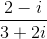The answer must be in standard form.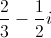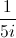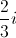Explanation:

Multiply both the numerator and the denominator by the conjugate of the denominator which is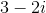which results in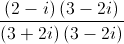The numerator after simplification give usThe denominator is equal toHence, the final answer in standard form =